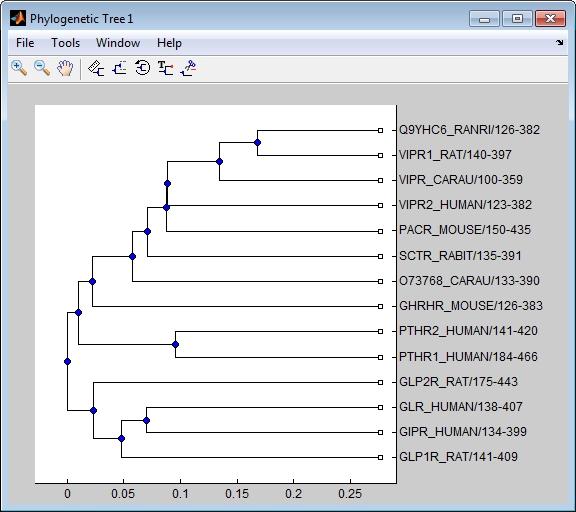# subtree (phytree)

Extract phylogenetic subtree

## Syntax

```Tree2 = subtree(Tree1, Nodes) ```

## Description

`Tree2 = subtree(Tree1, Nodes)` extracts a new subtree (`Tree2`) where the new root is the first common ancestor of the `Nodes` vector from `Tree1`. Nodes in the tree are indexed as `[1:NUMLEAVES]` for the leaves and as `[NUMLEAVES+1:NUMLEAVES+NUMBRANCHES]` for the branches. Nodes can also be a logical array of following sizes ```[NUMLEAVES+NUMBRANCHES x 1]```, `[NUMLEAVES x 1]` or ```[NUMBRANCHES x 1]```.

## Examples

1. Load a phylogenetic tree created from a protein family.

`tr = phytreeread('pf00002.tree');`
2. Get the subtree that contains the VIPR2 and GLR human proteins.

```sel = getbyname(tr,{'vipr2_human','glr_human'}); sel = any(sel,2); tr = subtree(tr,sel); view(tr)```## Version History

Introduced before R2006a